Question

# Write a python program using function prime_factorization(n) which will compute the product of all the prime...

Write a python program using function prime_factorization(n) which will compute the product of all the prime factors of a given input n.

```def is_prime(n):
if n > 1:
for i in range(2, n):
if (n % i) == 0:
return False
return n > 1

def prime_factorization(n):
product = 1
print("Prime factors of", n, "are:", end=' ')
for i in range(2, n + 1):
if is_prime(i) and n % i == 0:
product *= i
print(i, end=' ')
print()
return product

def main():
n = int(input("Enter an integer: "))
p = prime_factorization(n)
print("Product of prime factors of", n, "is", p)

main()
```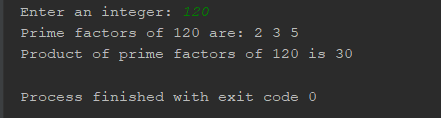#### Earn Coins

Coins can be redeemed for fabulous gifts.

Similar Homework Help Questions
• ### (Python) (15 points) Fundamental theorem of number theory states that every natural number n can be...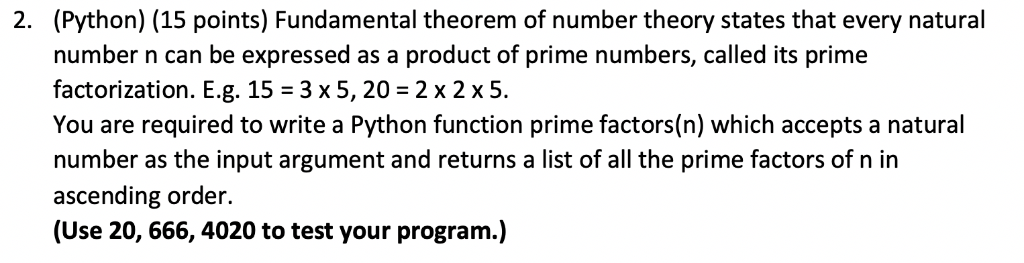(Python) (15 points) Fundamental theorem of number theory states that every natural number n can be expressed as a product of prime numbers, called its prime factorization. E.g. 15 3 x 5,20 2x 2x5. You are required to write a Python function prime factors(n) which accepts a natural number as the input argument and returns a list of all the prime factors of n in ascending order. (Use 20, 666, 4020 to test your program.) 2.

• ### How to solve it using Python? 5. Write a function called no_squares which takes an input...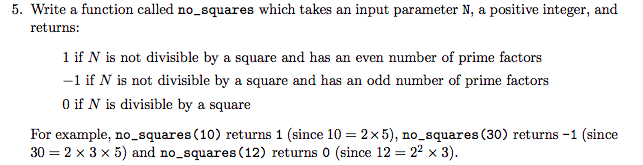How to solve it using Python? 5. Write a function called no_squares which takes an input parameter N, a positive integer, and returns: 1 if N is not divisible by a square and has an even number of prime factors -1 if N is not divisible by a square and has an odd number of prime factors 0 if N is divisible by a square For example, no-squares (10) returns 1 (since 10 = 2x5), no-squares (30) returns-1 (since 30...

• ### Your program  write a program to test whether a number is prime or not. Your program should...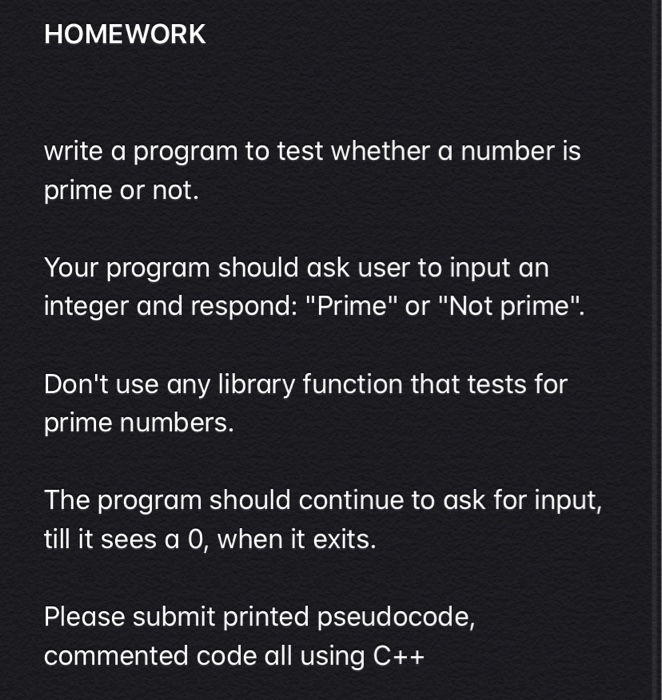Your program  write a program to test whether a number is prime or not. Your program should ask user to input an integer and respond: "Prime" or "Not prime". Don't use any library function that tests for prime numbers. The program should continue to ask for input, till it sees a 0, when it exits. Please submit printed pseudocodeshould ask user to input an integer and respond: "Prime" or "Not prime". Don't use any library function that tests for prime numbers....

• ### USING PYTHON 1. Write a small program that asks for an integer number from the user...

USING PYTHON 1. Write a small program that asks for an integer number from the user and print all the prime numbers (2,3,5,7,etc) less than the input number. 2. How long it takes for your program to print the prime numbers less than 100. (Use magic functions)

• ### PYTHON 3 PROGRAM PLEASE 18.19* (Prime number iterator) Write an iterator class for prime numbers. Invoking...

PYTHON 3 PROGRAM PLEASE 18.19* (Prime number iterator) Write an iterator class for prime numbers. Invoking the __next__() method returns the next prime number. Write a test program that displays all prime numbers less than 10000.

• ### USE PYTHON PLEASE Write a function called is prime which takes a single integer argument and...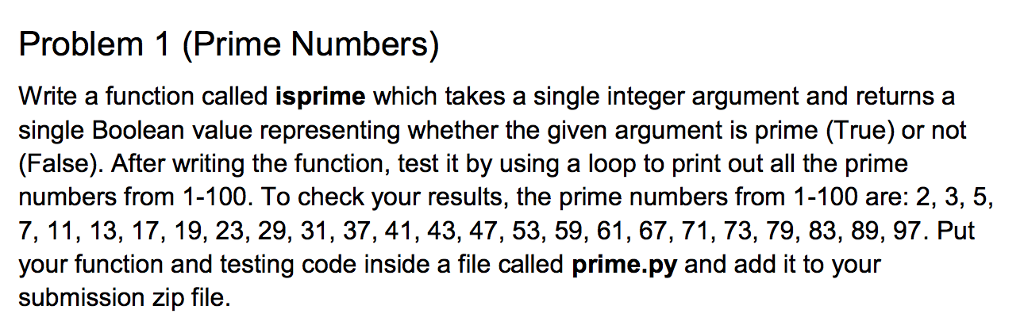USE PYTHON PLEASE Write a function called is prime which takes a single integer argument and returns a single Boolean value representing whether the given argument is prime (True) or not (False). After writing the function, test it by using a loop to print out all the prime numbers from 1-100. To check your results, the prime numbers from 1-100 are: 2, 3, 5, 7, 11. 13, 17, 19, 23, 29, 31, 37, 41, 43, 47, 53, 59, 61, 67,...

• ### Write a Python program that calculates the product of all odd numbers between 1 and 20...

Write a Python program that calculates the product of all odd numbers between 1 and 20 (1x3x5x7x9x11x13x15x17x19), using the for loop and the range function.

• ### Python Program 5. Write a Python program in a file named validTime.py. Include a function named...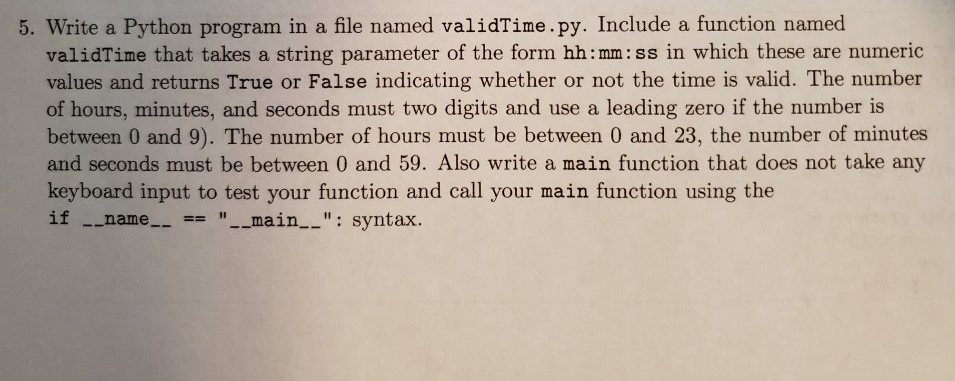Python Program 5. Write a Python program in a file named validTime.py. Include a function named string parameter of the form hh:mm: ss in which these are numeric validTime that takes a values and returns True or False indicating whether or not the time is valid. The number of hours, minutes, and seconds must two digits and use a between 0 and 9). The number of hours must be between 0 and 23, the number of minutes and seconds must...

• ### PYTHON: Using Lists and Arrays! 5. In a program, write a function that accepts two arguments:...

PYTHON: Using Lists and Arrays! 5. In a program, write a function that accepts two arguments: a list, and a number n. The program should generate 20 random numbers, in the range of 1 through 100, and assign each number to the list. The program should also ask the user to pick a number from 1 through a 100 and assign that number to n. The function should display all of the number in the list that are greater than...

• ### Lab 1 Q1. Write a Python program with the following function building block. •All input values...

Lab 1 Q1. Write a Python program with the following function building block. •All input values are to be taken fro m the user. •Ensure to check for possible error cases like mismatch of data type and input out of range, for every function.       Function 1: countVowels(sentence) – Function that returns the count of vowels in a sentence. Function 2: Sum of all even numbers between two numbers, identify number of parameters   Q2. Write a Python program that reads a...